## LCM and HCF

#### LCM and HCF

1. Find the greatest number which will divide 478 and 719 leaving remainders 2 and 5 respectively.

1. We can understand the problem in better way in this form,
i.e., (478 – 2) = 476 and (719 – 5) = 714 will be completely divisible by that number to be found.
For this to happen we take the HCF Of 476 and 714.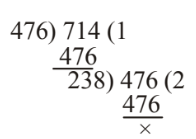##### Correct Option: D

We can understand the problem in better way in this form,
i.e., (478 – 2) = 476 and (719 – 5) = 714 will be completely divisible by that number to be found.
For this to happen we take the HCF Of 476 and 714.∴ Required number = HCF of 476 and 714 = 238

1. A wine seller had three types of wine, 403 litres of 1st kind, 434 litres of 2nd kind and 465 litres of 3rd kind. Find the least possible number of casks of equal size in which different types of wine can be filled without mixing.

1. For the least possible number of casks of equal size, the size of each cask must be of the greatest capacity. Hence, the capacity of the cask will be equal to the HCF of 403 l, 434 l and 465 l.
Now, HCF of 403 and 434.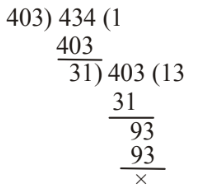Required HCF= HCF of 31 and 465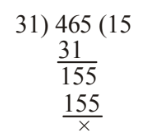∴ Required HCF = 31 litres = Capacity of a cask.

 ∴ required number of casks = 403 + 434 + 465 31 31 31

##### Correct Option: C

For the least possible number of casks of equal size, the size of each cask must be of the greatest capacity. Hence, the capacity of the cask will be equal to the HCF of 403 l, 434 l and 465 l.
Now, HCF of 403 and 434.Required HCF= HCF of 31 and 465∴ Required HCF = 31 litres = Capacity of a cask.

 ∴ required number of casks = 403 + 434 + 465 31 31 31

Hence , required number of casks = 13 + 14 + 15 = 42

1. Find the least number of square tiles by which the floor of a room of dimensions 16.58 × 8.32 m can be covered completely?

1. We require the least number of square tiles, hence each tile must be of maximum dimensions.
Hence, the maximum dimensions of a tile = HCF of 16.58 m and 8.32 m
Now, 16.58 m = 16.58 × 100 cm = 1658 cm
8.32 m = 8.32 × 100 cm = 832 cm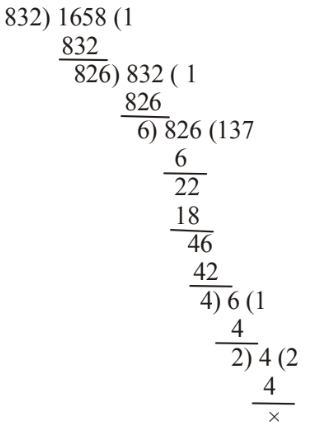Hence, the side of a square tile = 2 cm

 ∴ Required number of tiles = Area of floor Area of a square tile

##### Correct Option: B

We require the least number of square tiles, hence each tile must be of maximum dimensions.
Hence, the maximum dimensions of a tile = HCF of 16.58 m and 8.32 m
Now, 16.58 m = 16.58 × 100 cm = 1658 cm
8.32 m = 8.32 × 100 cm = 832 cmHence, the side of a square tile = 2 cm

 ∴ Required number of tiles = Area of floor Area of a square tile

 Required number of tiles = 1658 × 832 = 344864 2 × 2

1. A room is 26 metres long and 10 metres broad. Its floor is to be paved by square tiles. What will be the least number of tiles required to cover the floor completely ?

1. For the least number of tiles, each tile must be of maximum area.
Side of the largest tile = HCF of 26m and 10m
Side of the largest tile = HCF of 2 × 13m and 2 × 5 m = 2 metres { ∴ HCF of 26m and 10m = 2 }
∴ Area of a tile = 2 × 2 = 4 sq. metres

##### Correct Option: D

For the least number of tiles, each tile must be of maximum area.
Side of the largest tile = HCF of 26m and 10m
Side of the largest tile = HCF of 2 × 13m and 2 × 5 m = 2 metres { ∴ HCF of 26m and 10m = 2 }
∴ Area of a tile = 2 × 2 = 4 sq. metres

 ∴ The least number of tiles = Area of the floor = 26 × 10 = 65 Area of a tile 2 × 2

1. Three plots having an area of 165, 195 and 285 square metres respectively are to be sub-divided into equalised flower beds. If the breadth of a bed is 3 metres, what will the maximum length of a bed?

1. Maximum area of a bed = HCF of 165, 195 and 285.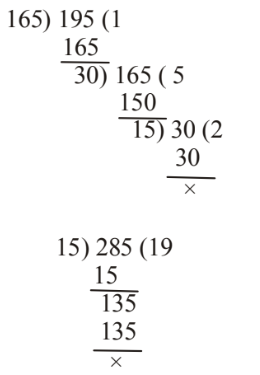∴ HCF = Maximum area = 15 sq. metres